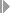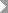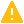Show TOC

###(11) Inventory Determination, Without Planned Costs, With Milestone Billing#### Use

You can use the method Inventory Determination, Without Planned Costs, With Milestone Billing for:

• Sales orders

• Projects

You can use the method Inventory Determination, Without Planned Costs, With Milestone Billing to:

• Calculate inventories in the amount of the actual costs if the actual revenue is zero

• Calculate the cost of sales in the amount of the actual costs of the period if the actual revenue is not zero

The inventory is canceled as soon as actual revenues are received. If actual costs are incurred in a subsequent period but no actual revenues are received, the system again creates inventory in the amount of the actual costs of the period.

Note the following:

• You cannot create reserves.

• You can use this method with milestone billing.

• There is no proportionality between cost of sales and revenue:

As soon as revenue is received, the actual costs are considered cost of sales in full. In other words, the cost of sales is not proportional to actual revenues as with the revenue-based method, nor is it proportional to the quantity sold as with the quantity-based method.

• You do not need any planned data.

• Sales and administration costs are reported as cost of sales.

#### Prerequisites

Choose a results analysis method in simplified Customizing for Product Cost by Sales Order underPeriod-End ClosingResults AnalysisValuation Method.

#### Features

R(PA) = R(a)

C(PA) = 0 if R(a) per = 0

If R(a) per <> 0 then C(PA) cum = C(a) cum

If R(a) per = 0 then C(z) = C(a) cum

Cum = cumulative values since the last actual revenue

Per = results analysis period

#### Example

Period 01

In period 01 you have actual costs of USD 20,000 but no revenues. In results analysis, the system calculates the following data:

• Cost of sales affecting net income of USD 0

• Revenue affecting net income of USD 0

• Capitalized costs of USD 20,000

You then settle the following:

• Capitalized costs to FI and EC-PCA

• No cost of sales and no revenues to CO-PA

The following values are reported in CO-PA:

Profitability Analysis

 Actual revenues 0 Calculated cost of sales 0 Profit 0

The income statement shows the following values:

Income Statement

 Expense Revenue Actual costs 20,000 Inventory increase Capitalized costs 20,000 20,000 20,000

Period 02

In period 02 actual costs increase to USD 80,000. You deliver to your customer and send an invoice for USD 100,000. The order is partially delivered and partially billed. In results analysis, the system calculates the following data:

• Cost of sales affecting net income of USD 80,000

• Revenue affecting net income of USD 100,000

• Capitalized costs of USD 0

You then settle the following:

• Cost of sales to CO-PA

• Revenues to CO-PA

• The cancellation of the capitalized costs to FI and EC-PCA

The following values are reported in CO-PA:

Profitability Analysis

 Revenues (actual revenues) 100,000 Cost of sales (actual costs) 80,000 Profit 20,000

The income statement shows the following values:

Income Statement

 Expense Revenue Actual costs 80,000 Actual revenues 100,000 Profit 20,000 100,000 100,000

Period 03

In period 03 actual costs increase to USD 90,000. No billing takes place. In results analysis, the system calculates the following data:

• Cost of sales affecting net income of USD 80,000

• Revenue affecting net income of USD 100,000

• Capitalized costs of USD 10,000

You then settle the following:

• Cost of sales to CO-PA

• Revenues to CO-PA

• The capitalized costs to FI and EC-PCA

The following values are reported in CO-PA:

Profitability Analysis

 Revenues (actual revenues) 100,000 Cost of sales (actual costs) 80,000 Profit 20,000Caution

This results analysis data was already settled to CO-PA in period 02.

End of the caution.

The income statement shows the following values:

Income Statement

 Expense Revenue Actual costs 90,000 Actual revenues 100,000 Profit 20,000 Capitalized costs 10,000 110,000 110,000

Period 04

In period 04 actual costs increase to USD 130,000. You deliver to your customer and send an invoice for USD 100,000. Total revenue is USD 200,000. The order is now fully delivered and fully invoiced. In results analysis, the system calculates the following data:

• Cost of sales affecting net income of USD 130,000

• Revenue affecting net income of USD 200,000

• Capitalized costs of USD 0

You then settle the following:

• Cost of sales to CO-PA

• Revenues to CO-PA

• The cancellation of the capitalized costs to FI and EC-PCA

The following values are reported in CO-PA:

Profitability Analysis

 Revenues (actual revenues) 200,000 Cost of sales (actual costs) 130,000 Profit 70,000

The income statement shows the following values:

Income Statement

 Expense Revenue Actual costs 130,000 Actual revenues 200,000 Profit 70,000 200,000 200,000

The profit on your sales order is USD 70,000.